# Dual transistor improves current-sense circuit

In multiple-output power supplies in which a single supply powers circuitry of vastly different current draws, two perplexing steps are sensing the current that each output draws and deactivating the power supply in the event of an overload on that output. These issues are especially important in protecting the fragile PCB traces in low-level circuits. A typical circuit would use the base-emitter threshold voltage of approximately 0.6 V of a bipolar transistor to trigger the power-supply-protection circuits. Although economical, the transistor’s threshold varies excessively over temperature; hence, the protection level is unstable.

The circuit in Figure 1 essentially eliminates the base-emitter-voltage temperature-variation problem as the derivation of the output voltage and as a function of the load current. By using dual bipolar devices in one case, the manufacturer nearly perfectly matches the two devices. Although this Design Idea describes a positive power supply, you can realize a similar negative-output-supply current-sense circuit using a dual NPN transistor in place of the dual PNP that the figure shows.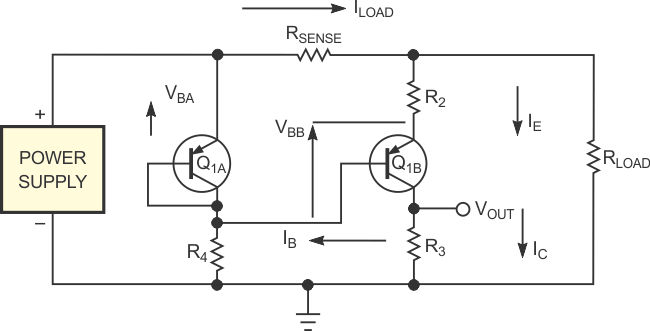Figure 1. This simple two-transistor circuit provides a voltage output proportional to the current through sense resistor RSENSE.

The following equations show the derivation of the output voltage as a function of the load current (referring to Figure 1):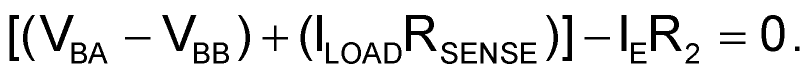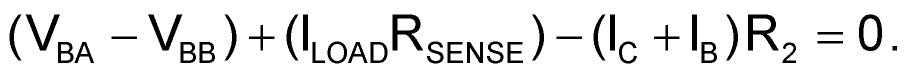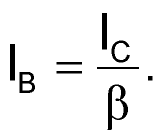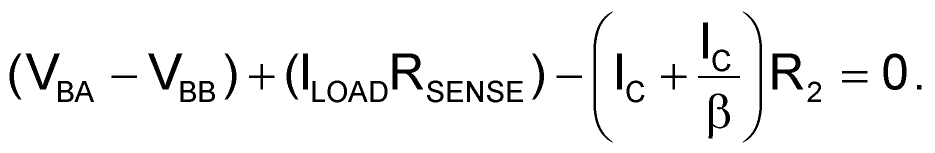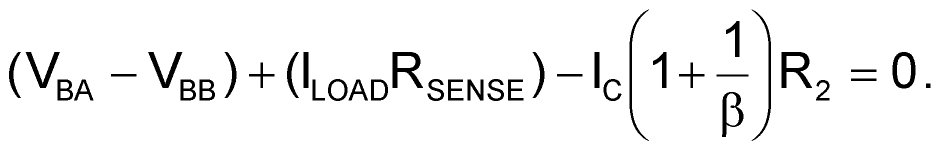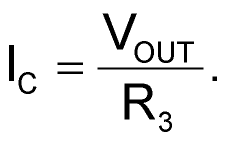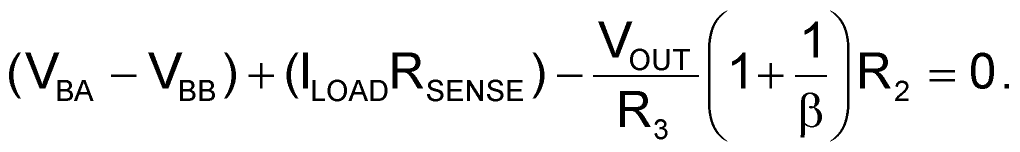If VBA = VBB, thenand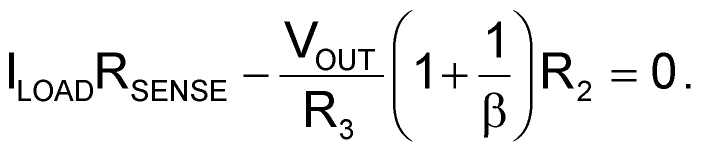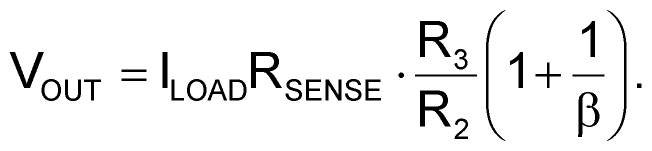If β is high, then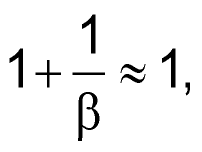and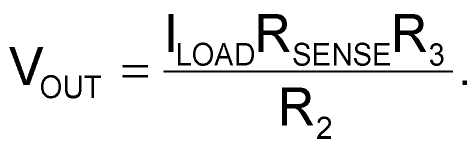EDN